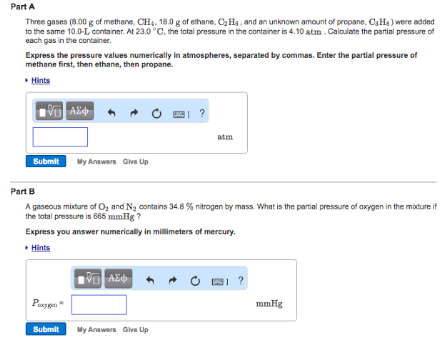# Problem: Part AThree gases (8.00 g of methane, CH4, 18.0 g of ethane, C2H4, and an unknown amount of propane, C3H8) were added to the same 10.0-L container. At 23.0°C, the total pressure in the container is 4.10 atm. Calculate the partial pressure of each gas in the container.Express the pressure values numerically in atmospheres, separated by commas. Enter the partial pressure of methane first, then ethane, then propane. Part B A gaseous mixture of O2 and N2 contains 34.8% nitrogen by mass. What is the partial pressure of oxygen in the mixture if the total pressure is 665 mmHg?Express you answer numerically in millimeters of mercury.

###### FREE Expert Solution
100% (399 ratings)###### Problem Details

Part A

Three gases (8.00 g of methane, CH4, 18.0 g of ethane, C2H4, and an unknown amount of propane, C3H8) were added to the same 10.0-L container. At 23.0°C, the total pressure in the container is 4.10 atm. Calculate the partial pressure of each gas in the container.

Express the pressure values numerically in atmospheres, separated by commas. Enter the partial pressure of methane first, then ethane, then propane.

Part B

A gaseous mixture of O2 and N2 contains 34.8% nitrogen by mass. What is the partial pressure of oxygen in the mixture if the total pressure is 665 mmHg?

Express you answer numerically in millimeters of mercury.